Next: CFQED Up: Commands Previous: LASER

## LASERQED

LASERQED COMPTONBREITWHEELER[,]NPH=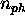, [NY=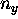,] [NXI=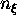,] [NLAMBDA=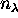,] [NQ=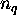,] XIMAX=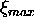, LAMBDAMAX=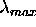, ETAMAX=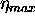, [PMAX=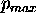,] [ENHANCEFUNCTION=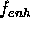,] ;
COMPTONBREITWHEELER
Specifies which parameters to define here.Maximum number of laser photons to be absorbed in one process.
If <0, turn off Compton or Breit-Wheeler.
If =0, use linear Compton/Breit-Wheeler formula.
If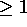, use nonlinear formula.
Note that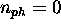and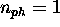are different. The former is the limit of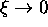, which containsterm only, where as the latter is a truncation of the exact series with respect to. When, none of the following variables are needed.
When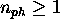, only longitudinal polarization is considered and the lasers must be circularly polarized by 100% (i.e.,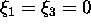,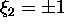).Number of abscissa for final energy. Default=20.Number of abscissa for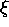parameter. Default=20.Number of abscissa for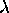parameter. Applies to Compton case only. Default=20.Number of abscissa for q parameter. Applies tp Breit-Wheeler case only. Default=50.Maximum value offor the table.Maximum value offor the table. Applies to Compton case only.Maximum value of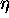for the table. Applies to Breit-Wheeler case only.Maximum probability of events per one time step. If the computed probability exceeds, CAIN of present version stops with a message.Defines a function in order to enhance a part of spectrum.   It is defined as an expression containing Y as the final energy parameter (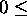Y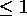). Its value must beforY. Generally speaking, Y close to 1 generates low energy charged particles. For example,
E NH=1+Step(Y-0.8)*(Y-0.8)*10
will enhance the events with Y>0.8 by a factor upto 3 (at Y=1).
In the program, the real spectrum function is multiplied byand, when an event is generated, the created particles are asigned a weight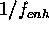.
Note thatslightly larger than 1 is useless (even harmful) because a small fraction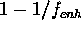of the parent particle will remain as a macro-particle, causing a waste of computing time. In the example above,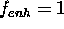exactly for Y<0.8.
This function is used only during the initialization by LASERQED command. Therefore, if the expression contains user-defined parameters, their values at the time of LASERQED command are used. Changing them afterwards will not affect the computation.

See Sec.5.7.3 and Appendix.A for more detailNext: CFQED Up: Commands Previous: LASER

Toshiaki Tauchi
Thu Dec 3 17:27:26 JST 1998